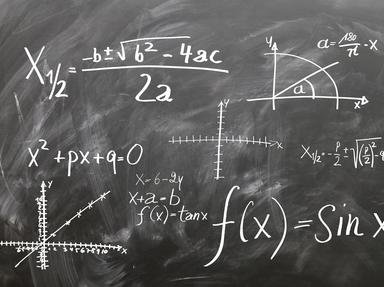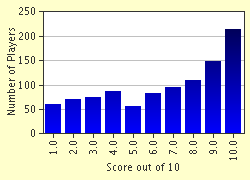# Find that Number! Trivia Quiz

### Based on information provided to you about a number, you have to say what that number is. Though a pencil and paper might be helpful, they aren't strictly necessary. Enjoy!

A multiple-choice quiz by achernar. Estimated time: 4 mins.

Author
achernar
Time
4 mins
Type
Multiple Choice
Quiz #
200,611
Updated
Dec 03 21
# Qns
10
Difficulty
Average
Avg Score
6 / 10
Plays
7777
Last 3 plays: Guest 112 (0/10), TERRYROYHURST (0/10), Guest 76 (1/10).
This quiz has 2 formats: you can play it as a or as shown below.
Scroll down to the bottom for the answer key.
1. A natural number is doubled when added to its reciprocal. Find that number! Hint

1/2
2
1
0

#### NEXT>

2. The logarithm (base ten) of five hundred times an integer is three. Find that number! Hint

2
1500
450
8

#### NEXT>

3. A natural number is halved when reduced by thirteen. Find that number!

#### NEXT>

4. Twice a negative integer is eight less than its square. Find that number! Hint

16
8
-2
-64

#### NEXT>

5. A positive integer is equal to 4 times its reciprocal. Find that number! Hint

2
3
-4
9

#### NEXT>

6. The sum of the square of a negative integer and itself is zero. Find that number! Hint

-1
-2
0
-1/2

#### NEXT>

7. The product of a positive integer and the integer that succeeds it is twelve. Find that number! Hint

5
3
26
27

#### NEXT>

8. The digit in the units place of a two-digit number exceeds that in the tens place by one. If the number is equal to four times the sum of its digits, find that number!

#### NEXT>

9. A number added to twice itself gives twenty-one. Find that number!

#### NEXT>

10. The fourth power of a positive integer is equal to four times its square. Find that number! Hint

2
1
0
4

 (Optional) Create a Free FunTrivia ID to save the points you are about to earn:Select a User ID:Choose a Password:Your Email:

Quiz Answer Key and Fun Facts
1. A natural number is doubled when added to its reciprocal. Find that number!

Let the number be "x". This gives:

x + 1/x = 2x
=> 1/x = 2x - x
=> 1/x = x
=> x^2 = 1
=> x = [plus or minus] 1

Since x is a natural number, it has to be positive; therefore x = 1.

===
(In case you didn't know, the carat (^) sign is used before exponential powers, e.g., "seven cubed" is written as "7^3".)
2. The logarithm (base ten) of five hundred times an integer is three. Find that number!

Let the unknown number be "x".

log(500x) = 3

Notice that 3 can be written as "log(1000)", because 10^3 = 1000. Substituting "3" with "log(1000)",

log(500x) = log(1000)

Cancelling log from both sides,

500x = 1000
=> x = 2

And so the value of the integer is 2.
3. A natural number is halved when reduced by thirteen. Find that number!

Let the number be "x". (Pardon me for using "x" each time to represent the unknown quantity, just don't want to deviate from tradition.)

x - 13 = (1/2)x
=> x - (1/2)x = 13
=> (2x - x)/2 = 13
=> x/2 = 13
=> x = 26
4. Twice a negative integer is eight less than its square. Find that number!

Let the number be "x". According to the given data:

2x = x^2 - 8
=> x^2 - 2x - 8 = 0
=> x^2 - 4x + 2x - 8 = 0
=> x(x - 4) + 2(x - 4) = 0
=> (x + 2)(x - 4) = 0

This gives x = -2 or x = 4. However, as it's a negative integer we're looking for, 4 cannot be the answer, which means it is -2.
5. A positive integer is equal to 4 times its reciprocal. Find that number!

Let tbe positive integer be "x". This means its reciprocal is (1/x).

x = 4(1/x)
=> x = 4/x
=> x^2 = 4
=> x = 2 or -2

Since "x" is supposed to be positive, the number is 2.
6. The sum of the square of a negative integer and itself is zero. Find that number!

Let the negative integer be "x".

x^2 + x = 0
=> x(x + 1) = 0
=> x = 0 or -1

As we're looking for a negative integer, -1 is the answer.
7. The product of a positive integer and the integer that succeeds it is twelve. Find that number!

Let the unkown number be "x". Therefore, the number that succeeds it is (x + 1). According to what's given,

x(x + 1) = 12
=> x^2 + x = 12
=> x^2 + x - 12 = 0

Factorising the left hand side of this quadratic equation,

(x - 3)(x + 4) = 0

This gives x = 3 or -4. However, since it's a positive integer we're looking for, we reject x = -4, thus leaving only x = 3 as a possible answer.
8. The digit in the units place of a two-digit number exceeds that in the tens place by one. If the number is equal to four times the sum of its digits, find that number!

Let the digit in the tens place be "x". Since the digit in the units place exceeds that in the tens place by 1, it becomes (x + 1).

The sum of the digits = x + x + 1 = 2x + 1

Any two-digit number can be expressed in the following manner: 10*(digit in the tens place) + (digit in the units place)
e.g. 34 = 10*3 + 4; 71 = 10*7 + 1

Thus, the number we are seeking can be expressed as [10x + (x + 1)].

According to the given data,

4(2x + 1) = 10x + (x + 1)
=> 8x + 4 = 10x + x + 1
=> 3x = 3
=> x = 1

Hence, the digit in the tens place is 1. The digit in the units place is given by (x + 1) = 1 + 1 = 2.

And so the number is 12.
9. A number added to twice itself gives twenty-one. Find that number!

Let the number be "x".

x + 2x = 21
=> 3x = 21
=> x = 7

Whoever said algebra was tough? :-)
10. The fourth power of a positive integer is equal to four times its square. Find that number!

Let the number be "x". This gives:

x^4 = 4x^2
=> (x^2)^2 = 4(x^2)

To make things simple, let x^2 = y.

=> y^2 = 4y
=> y^2 - 4y = 0
=> y(y - 4) = 0
=> y = 0 or 4

If y = 0 then x = 0 and if y = 4 then x = 2 (because two squared equals four). Since it is given that x is a positive integer (which 0 isn't), the number is 2.

===
I hope this quiz helped exercise your grey matter and you had a good time playing it!
Source: Author achernar

This quiz was reviewed by FunTrivia editor crisw before going online.
Any errors found in FunTrivia content are routinely corrected through our feedback system.
Most Recent Scores
Jan 16 2023 : Guest 112: 0/10
Jan 02 2023 : TERRYROYHURST: 0/10
Dec 23 2022 : Guest 76: 1/10
Dec 20 2022 : Vendetta125: 7/10

Score DistributionRelated Quizzes